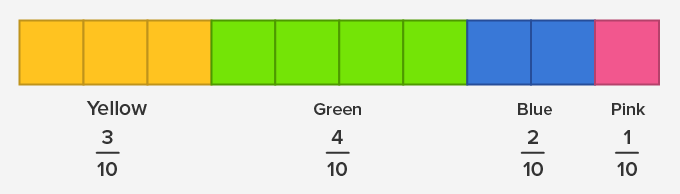# Subtracting Fractions - Definition with Examples

The Complete K-5 Math Learning Program Built for Your Child

• 40 Million Kids

Loved by kids and parent worldwide

• 50,000 Schools

Trusted by teachers across schools

• Comprehensive Curriculum

Aligned to Common Core

## Subtracting fractions

Like fractions

Fractions with the same denominators are called like fractions.

For instance:

Example 1-In the picture below, all the fractions are like fractions.Unlike fractions

Fractions with different denominators are known as unlike fractions.

Subtracting fractions

It is easier to add or subtract like fractions because either they are equal parts from the same whole or they are the parts of the same size.

Case 1- Subtracting like fractions

• Subtract the numerator and it will give us the answer.
• Simplify the fraction, if required.

For instance:

Example 2- Subtract from 3 15  from 12 15 .

Solution: As both, the fractions are like fractions

∴ 1215-315 = 12-3 15 = 9 15

As 9 15  can be simplified, ∴ the final answer is 3 5 .

Case 2- Subtracting unlike fractions

Below are the steps to subtract unlike fractions:

• Find the least common multiple (LCM) of the denominators.
• Change the denominator to the LCM by multiplying the numerator and the denominator by the same number.
• Once the fractions have the same denominators, subtract the numerators of the fractions.
• The difference between the numerator and the LCM will be the numerator and the denominator of the answer respectively.
• Simplify the fraction, if required.
• For instance:

Example 3- Subtract 4 7 from  3 5 .

Solution – We have to find, 3 5 - 4 7

As both the fractions are unlike fractions (different denominators), we need to make the denominators the same for subtraction.

The least common multiple of 7 and 5 is 35 .

To make the denominators 35, the fraction 3 5 can be written as 3x7 5x7 = 21 35  and the fraction 4 7 can be written as 4x5 7x5 = 20 35

Now, the question can be rewritten as 21 35 - 20 35 .

As the denominators are the same, &there  21 35 - 20 35 = 1 35

Since, 1 35  is in the simplest form, therefore the final answer is  1 35  .

 Fun Facts: We cannot add or subtract fractions without converting them into a like fraction.

Won Numerous Awards & Honors